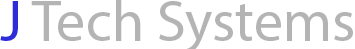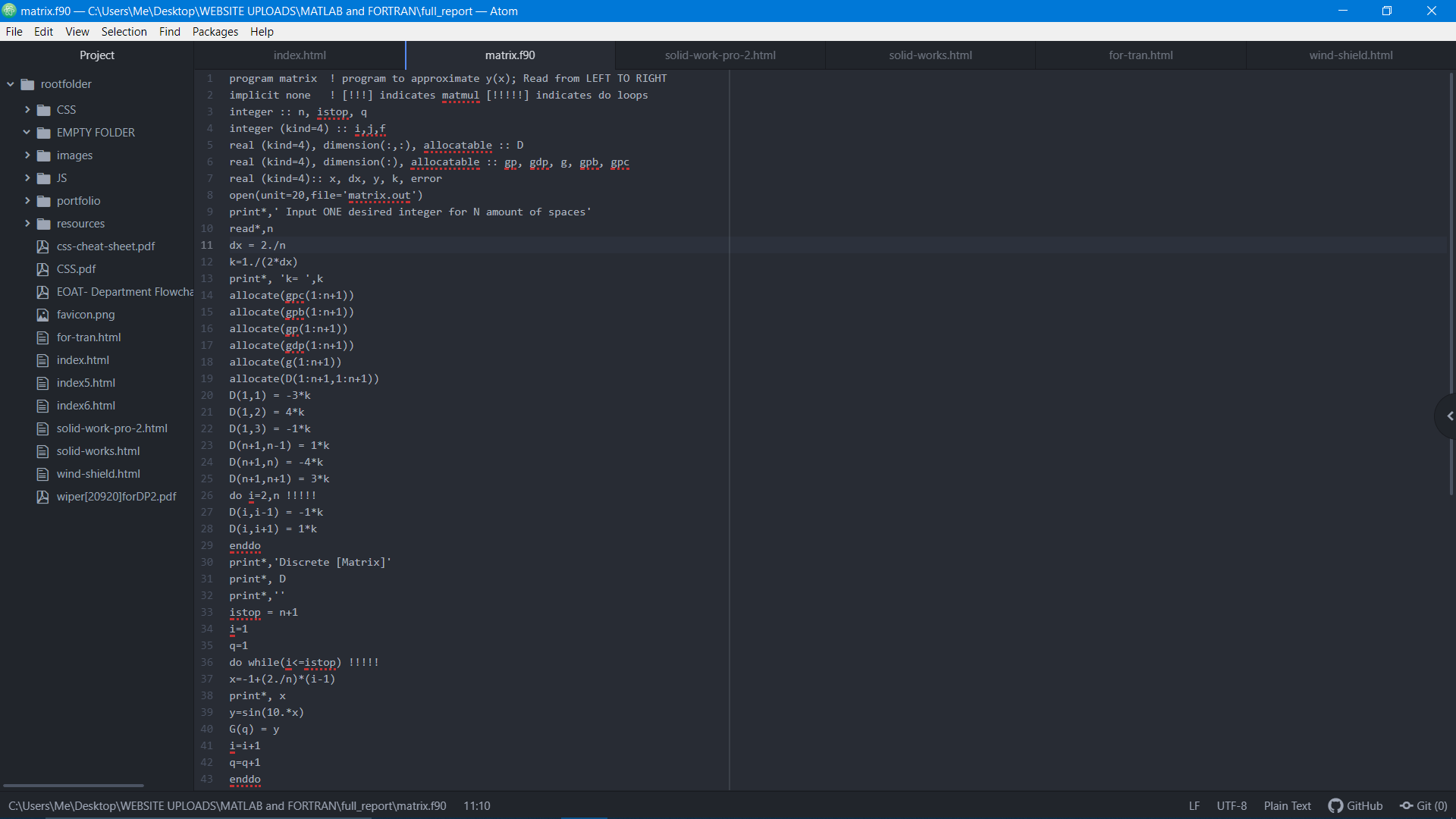## Collaboration is key

Together we can face any challenges as deep as the ocean and as high as the sky.
-Sonia Ghandi

Interested in working with me? I enjoy working with a team of engineers developing new projects for world-wide access.

## Overview:

This is a Fortran program that estimates the derivative of a function based on its known value at discrete points. The technique that helps utilizes this program is called the central difference. This method required the first and second derivatives of a known function in order to capture the value at uniformly distributed points. An error assessment was also implemented to determine how the magnitude error in the first derivative at one arbitrary point varies as the initial values of the dependent variable changes. A technical paper written in LaTeX describes the purpose of this Fortran project as well as its results.## Programming Languages Used:

• Fortran
• MATLAB 2018

Fortran was used as the main program that complies the data into a single text document. That same text document was read into MATLAB in order to develop graphical plots.Go back to projects